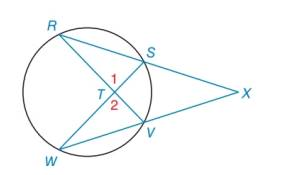Chapter 6.2, Problem 33EElementary Geometry For College St...

7th Edition
Alexander + 2 others
ISBN: 9781337614085

Solutions

Chapter
SectionElementary Geometry For College St...

7th Edition
Alexander + 2 others
ISBN: 9781337614085
Textbook Problem

In the figure shown, Δ R X V ∼ Δ W X S by the reason A A . Name two pairs of congruent angles in these similar triangles.Exercises 32, 33

To determine

To name:

The two pairs of congruent angles in the given similar triangles.

Explanation

Calculation:

Given, ΔRXVΔWXS by the reason of AA similarity.

Consider the figure,

By the vertical angle theorem,

1=2.

Therefore, from the figure, RW

Still sussing out bartleby?

Check out a sample textbook solution.

See a sample solution

The Solution to Your Study Problems

Bartleby provides explanations to thousands of textbook problems written by our experts, many with advanced degrees!

Get Started

Describe the scores in a sample that has standard deviation of zero.

Statistics for The Behavioral Sciences (MindTap Course List)

Solve the equations in Exercises 126. x4x2=6

Finite Mathematics and Applied Calculus (MindTap Course List)

True or False: If f(x) = g(x) for all x then f(x) = g(x).

Study Guide for Stewart's Single Variable Calculus: Early Transcendentals, 8th